# Class 11 Maths NCERT Solutions for Chapter 10 Straight Lines Exercise 10.1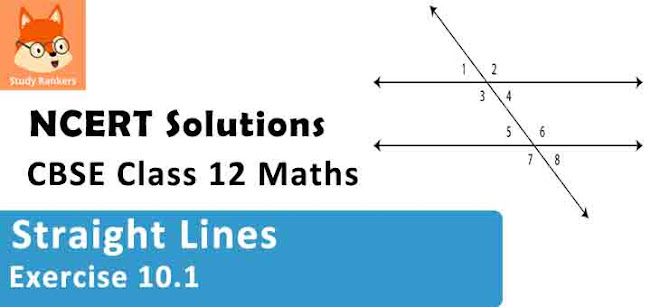### Straight Lines Exercise 10.1 Solutions

1. Draw a quadrilateral in the Cartesian plane, whose vertices are (–4, 5), (0, 7), (5, –5) and (–4, –2). Also, find its area.

Solution

Let ABCD be the given quadrilateral with vertices A (–4, 5), B (0, 7), C (5, –5), and D (–4, –2).
Then, by plotting A, B, C, and D on the Cartesian plane and joining AB, BC, CD, and DA, the given quadrilateral can be drawn as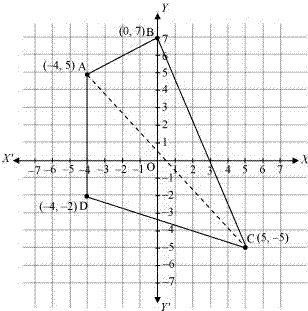To find the area of quadrilateral ABCD, we draw one diagonal, say AC.
Accordingly, area (ABCD) = area (ΔABC) + area (ΔACD)
We know that the area of a triangle whose vertices are (x1y1), (x2y2), and (x3y3) is
½ |x1 (y2 – y3 ) + x2 (y3 – y1 ) + x3 (y1 – y2 )|
Therefore, area of ΔABC
= 1/2 |-4(7+5) + 0(-5-5) + 5(5 - 7)| unit2
= 1/2 |-4(12)+ 5(-2)| unit2
= 1/2 |-48 - 10| unit2
= 1/2 |-58| unit2
= 1/2 × 58 unit2
= 29 unit2
Area of Δ ACD
= 1/2 |-4(-5 + 2) + 5(-2 - 5) + (-4)(5 + 5)| unit2
= 1/2 |-4(-3) + 5(-7) - 4(10)| unit2
= 1/2 |12 - 35 - 40| unit2
= 1/2 |-63| unit2
= 63/2 unit2
Thus, area (ABCD) = (29 + 63/2) unit2 = (58 + 63)/2 unit2 = 121/2 unit2

2. The base of an equilateral triangle with side 2a lies along they y-axis such that the mid point of the base is at the origin. Find vertices of the triangle.

Solution

Let ABC be the given equilateral triangle with side 2a.
Accordingly, AB = BC = CA = 2a
Assume that base BC lies along the y-axis such that the mid-point of BC is at the origin.
i.e., BO = OC = a, where O is the origin.
Now, it is clear that the coordinates of point C are (0, a), while the coordinates of point B are (0, –a).
It is known that the line joining a vertex of an equilateral triangle with the mid-point of its opposite side is perpendicular.
Hence, vertex A lies on the y-axis.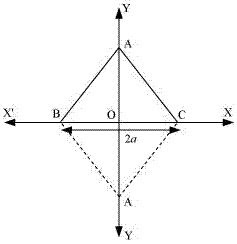On applying Pythagoras theorem to ΔAOC, we obtain
(AC)2 = (OA)2 + (OC)2
⇒ (2a)2 = (OA)2 + a2
⇒ 4a2 – a2 = (OA)2
⇒ (OA)2 = 3a2
⇒ OA = √3a
∴ Coordinates of point A = (± √3a, 0)
Thus, the vertices of the given equilateral triangle are (0, a), (0, -a) and (√3a, 0) or (0, a) , (0, -a) and (-√3a, 0).

3. Find the distance between P (x1, y1) and Q (x2, y2) when : (i) PQ is parallel to the y-axis, (ii) PQ is parallel to the x-axis

Solution

The given points are P(x1, y1) and Q (x2, y2).
(i) When PQ is parallel to the y - axis, x1 = x2.
In this case, distance between P and Q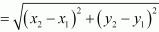= √(y2 – y1)2
= |y2 – y1|

(ii) When PQ is parallel to the x - axis, y1 = y2.
In this case, distance between P and Q= √(x2 – x1)2
= |x2 – x1|

4. Find a point on the x-axis, which is equidistant from the points (7, 6) and (3, 4).

Solution

Let (a, 0) be the point on the x axis that is equidistant from the points (7, 6) and (3, 4).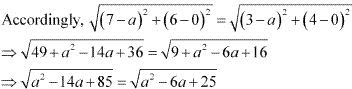On squaring both sides, we obtain
a2 – 14a + 85 = a2 – 6a + 25
⇒ –14a + 6a = 25 – 85
⇒ –8a = –60
⇒ a = 60/8 = 15/2
Thus, the required point on the x - axis is (15/2, 0)

5. Find the slope of a line, which passes through the origin, and the mid-point of the line segment joining the points P (0, –4) and B (8, 0).

Solution

The coordinates of the mid-point of the line segment joining the points
P (0, –4) and B (8, 0) are [(0+8)/2 , (-4+0)/2] = (4, -2)
It is known that the slope (m) of a non - vertical line passing through the points (x1, y1) and (x2, y2) is given by m = (y2 – y1 )/(x2 – x1 ) , x2 ≠ x1 .
Therefore, the slope of the line passing through (0, 0) and (4, -2) is
(-2-0)/(4 -0) = -2/4 = -1/2
Hence, the required slope of the line is -1/2.

6. Without using the Pythagoras theorem, show that the points (4, 4), (3, 5) and (–1, –1) are the vertices of a right angled triangle.

Solution

The vertices of the given triangle are A (4, 4), B (3, 5), and C (–1, –1).
It is known that the slope (m) of a non-vertical line passing through the points (x1, y1) and (x2, y2) is given by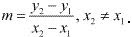∴ Slope of AB(m1) = (5 - 4)/(3 -4) = -1
Slope of BC(m2 ) = (-1-5)/(-1-3) = -6/-4 = 3/2
Slope of CA(m3 ) = (4 + 1)/(4+1) = 5/5  = 1
It is observed that m1m3 = -1
This shows that line segments AB and CA are perpendicular to each other
i.e., the given triangle is right-angled at A (4, 4).
Thus, the points (4, 4), (3, 5), and (–1, –1) are the vertices of a right-angled triangle.

7. Find the slope of the line, which makes an angle of 30° with the positive direction of y-axis measured anticlockwise.

Solution

If a line makes an angle of 30° with the positive direction of the y-axis measured anticlockwise, then the angle made by the line with the positive direction of the x-axis measured anticlockwise is 90° + 30° = 120°.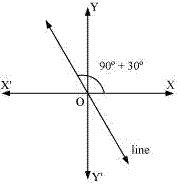Thus, the slope of the given line is tan 120° = tan(180° - 60°) = -tan 60° = -√3

8. Find the value of x for which the points (x, –1), (2, 1) and (4, 5) are collinear.

Solution

If points A(x, -1), B(2, 1) and C(4, 5) are collinear, then
Slope of AB = Slope of BC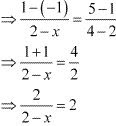⇒ 2 = 4 - 2x
⇒ 2x = 2
⇒ x = 1
Thus, the required value of x is  1.

9. Without using distance formula, show that points (–2, –1), (4, 0), (3, 3) and (–3, 2) are vertices of a parallelogram.

Solution

Let points (–2, –1), (4, 0), (3, 3), and (–3, 2) be respectively denoted by A, B, C, and D.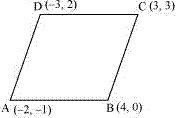Slope of AB = (0+1)/(4 +2) = 1/6
Slope of CD = (2 -3)/(-3-3) = -1/-6 = 1/6
⇒ Slope of AB = Slope of CD
⇒ AB and CD are parallel to each other.
Now, slope of BC = (3 -0)/(3 - 4) = 3/-1 = -3
Slope of AD = (2 +1)/(-3+2) = 3/-1 = -3
⇒ Slope of BC = Slope of AD
⇒ BC and AD are parallel to each other.
Therefore, both pairs of opposite sides of quadrilateral ABCD are parallel. Hence, ABCD is a parallelogram.
Thus, points (-2, -1), (4, 0), (3, 3) and (-3, 2) are the vertices of a parallelogram.

10. Find the angle between the x-axis and the line joining the points (3, –1) and (4, –2).

Solution

The slope of the lien joining the points (3, -1) and (4, -2) is m = [(-2-(-1)]/(4 -3) = -2 +1 = -1
Now, the inclination (θ ) of the line joining the points (3, –1) and (4, – 2) is given by
tan θ = –1
⇒ θ = (90° + 45°) = 135°
Thus, the angle between the x-axis and the line joining the points (3, –1) and (4, –2) is 135°

11. The slope of a line is double of the slope of another line. If tangent of the angle between them is 1/3, find the slopes of the lines .

Solution

Let m1 and m be the slopes of the two given lines such that m1 = 2m .
We know that if θ is the angle between the lines l1  and l2 with slopes m1 and m2 , then
θ = |(m2 - m1 )/(1 + m1 m2 )|.
It is given that the tangent of the angle between the two lines is 1/3.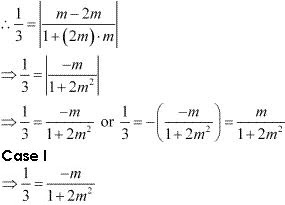⇒ 1 + 2m2 = -3m
⇒ 2m2 + 3m + 1 = 0
⇒ 2m2 + 2m + m + 1 = 0
⇒ 2m(m + 1) + 1(m + 1) = 0
⇒ (m + 1)(2m + 1) = 0
⇒ m = -1 or m = -1/2
If m = -1, then the slopes of the lines are  -1 and -2.
If m = -1/2, then the slopes of the lines are  -1/2 and -1.

Case II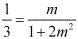⇒ 2m2 + 1 = 3m
⇒ 2m2 - 3m + 1 = 0
⇒ 2m2 - 2m - m + 1 = 0
⇒ 2m (m - 1) - 1(m - 1) = 0
⇒ (m - 1)(2m - 1) =0
⇒ m = 1 or m = 1/2
If m = 1, then the slopes of the lines are  1 and  2.
If m = 1/2, then the slopes of the lines are 1 and 2.
If m = 1/2, then the slopes of the lines are 1/2 and  1.
Hence, the slopes of the lines are -1 and -2 or -1/2 and -1 or 1 and  2 or 1/2 and 1.

12. A line passes through (x1, y1) and (h, k). If slope of the line is m, show that k – y1 = m (h – x1).
Solution
The slope of the line passing through (x1, y1) and (h, k) is (k - y1 )/(h - x1 ) .
It is given that the slope of the line is m.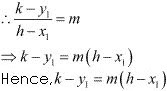13. It three point (h, 0), (a, b) and (0, k) lie on a line, show that a/h + b/k = 1 .
Solution
If the points A(h, 0), B(a, b), and C(0, k) lie on a line, then
Slope of AB = Slope of BC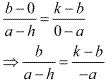⇒ -ab = (k - b)(a - h)
⇒ -ab = ka - kh - ab +  bh
⇒ ka + bh = kh
On dividing both sides by kh, we obtain
ka/kh + bh/kh = kh/kh
⇒ a/h + b/k = 1
Hence, a/h + b/k = 1

14. Consider the given population and year graph. Find the slope of the line AB and using it, find what will be the population in the year 2010?
Solution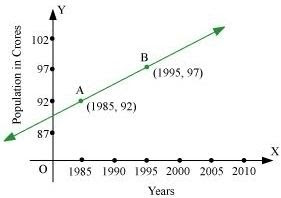Solution
Since line AB passes trough points A(1985, 92) and B(1995, 97), its slope is (97 - 92)/(1995 - 1985) = 5/10 = 1/2
Let y be the population in the year 2010. Then, according to the given graph, line AB must pass through point C(2010, y) :
∴ Slope of AB = Slope of BC.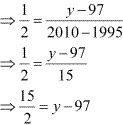⇒ y - 97 = 7.5
⇒ y = 7.5 + 97 = 104.5
Thus, the slope of line AB is 1/2 , while in the year 2010, the population will be 104.5 crores